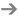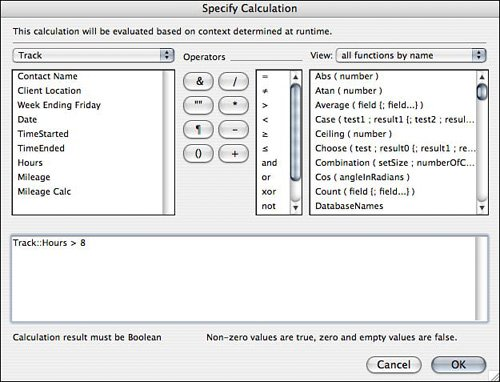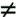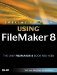# Understanding How and Where Calculations Are Used

Calculation functions are among the most important and powerful tools at your disposal in the development of FileMaker Pro solutions. Some people find learning calculations to be an easy task, whereas others can find writing complex calculations to be daunting. Whichever camp you fall into, calculations will enable you to unlock much of the advanced power within FileMakerwe encourage you to stick with it. Our hope is that this chapter and its companion, Chapter 14, "Advanced Calculation Techniques," will provide you a solid grounding.

This chapter focuses on basic calculation functions and techniques for using them well. Chapter 14 looks at more advanced calculation formulas and specific techniques. If you're new to FileMaker, you should start here. Those who have been using FileMaker for years may want to just skim this chapter. (There are probably a few nuggets of information that will make it worth your while.) We've also included a complete function reference in our companion book, FileMaker 8 Functions and Scripts Desk Reference. That book is intended to serve as a reference when you need to look up specific information. Here, we take more of a tutorial approach and explore how and why one employs calculations.

From the outset, it's important to understand the difference between calculation fields and calculation formulas. The term calculation is often used ambiguously to denote both concepts ("That table has more than 100 calculations!" or "What's the calculation used to determine access to this record?"). Calculation fields are a particular type of field whose value is determined through the evaluation of a calculation formula. Calculation formula is a broader concept that refers to any use of a formula to determine an output. When you learn "calculations," you're really learning calculation formulas. It so happens that you'll use calculation formulas to construct calculation fields, but the formulas are applied widely throughout FileMaker solutions.

Writing Calculation Formulas

Essentially, the purpose of a calculation formula is to evaluate an expression and return a value. In Figure 8.1, for example, you can see the field definition for a calculation field called Mileage Calc. The value of this field is defined to be the result of multiplying the contents of the Mileage field by .37, which is a typical mileage reimbursement rate.

Figure 8.1. When defining calculation fields, you specify an expression to evaluate in the Specify Calculation dialog.Most of the expressions you use in calculation formulas are intended to return a value, and that value might be a number, a text string, a date or time, or even a reference to a file to place in a container field. Another class of formulas, however, is intended to evaluate the veracity of an equation or statement. The value returned by these formulas is either a 1, indicating that the equation or statement is true, or 0, indicating that the equation or statement is false. Typically, calculations are used in this manner in If script steps, in calculated validations, and for defining field access restrictions.To learn more about field validation, see Chapter 3, "Defining and Working with Fields," p. 67.

In Figure 8.2, for instance, you can see a calculation dialog that specifies the condition for an If script step. When the script executes, FileMaker evaluates whether the number of hours is in fact greater than 8 (based on the current record's data). Depending on the value of the Hours field for any particular record, the statement may be either true or false and the script will presumably react accordingly.

Figure 8.2. Calculation formulas are often used to determine the truthfulness of an equation or a statement.For certain uses, then, the purpose of a calculation formula is to return a value; for other uses it's simply to evaluate the veracity of an equation or statement. In situations in which FileMaker is expecting a formula that returns a true/false result, you see the words Calculation result must be Boolean near the bottom of the calculation dialog. The If script step shown earlier is a typical example of this situation. Boolean is a software programming term for a value with one of two states: true or false. Any value returned other than 0 or a null value (for example, an empty string) is considered true.

Note

Note that Boolean rules apply for text values, dates, negative numbers, and so on. "Hello" is true (not zero and not null), a single space character (" ") is true, and -1 is true. Note also that the results of a formula are evaluated in the same way: (0 * 100) is false. (0 + 100) is true. Last, also note that you can use comparative operators: 1 and 1 is true (where each clause on both sides of the and operator evaluate to true), 1 or 1 is true, 1 xor 1 is false, and so on. You'll learn about operators later in the chapter.

Caution

The GetAsBoolean() function treats all data as numeric, such that, for example, "hello" evaluates as false and "hello999" evaluates as true. This is an inconsistency with the way in which other Boolean logic operates, so be sure to take note of it.

Uses for Calculation Formulas

This chapter focuses on the use of calculation formulas in field definitions, but it's important that you understand that there are other places where calculation formulas are used as well. Briefly, these include the following:

• Script steps Calculation formulas come into play in many script steps. The If, Set Field, and Set Variable script steps are notable examples. Many other script steps allow you to use a calculation formula to act as a parameter. A sampling includes Go to Layout, Go to Field, Go to Record, Pause/Resume Script, and Omit Multiple. Additionally, calculation formulas can be used to define script parameters and script results.
• Field validation One of the options available to you for validating data entry is validating by calculation. This, in effect, lets you define your own rules for validation. For example, you might want to test that a due date falls on a weekday, or perhaps that a status field not allow a value of "complete" if there is data missing elsewhere in a record.

The equation you provide is evaluated every time a user modifies the field. If it evaluates as true, the user's entry is committed. If it doesn't, the user is presented with an error message. For instance, if a user is supposed to enter a callback date on a contact record, you might want to validate that the entry is a future date. To do this, you might use the formula Call_Back_Date > Get ( CurrentDate ) as the validation for the Call_Back_Date field.

• Record-level security When you define privilege sets, you have the option of limiting a user's access to view, edit, and delete records based on a calculation formula you provide. If the equation you provide evaluates as true, the user can perform the action; if not, the action is prohibited. For instance, you might want to prevent users from inadvertently modifying an invoice that has already been posted. So you'd set up limited access for editing records based on the formula Invoice_Status"Posted. Only records for which that is a true statement would be editable.
• Auto-entry options When you're defining text, number, date, time, and timestamp fields, several auto-entry options are available for specifying default field values. One of these options is to auto-enter the result of a calculation formula. For instance, in a contact management database, you might want a default callback date set for all new contact records. The formula you'd use for this might be something like Get ( CurrentDate ) + 14, if you wanted a callback date two weeks in the future.
• Calculated replace A calculated replace is a way of changing the contents of a field in all the records in the current found set. It's particularly useful for cleaning up messy data. Say, for example, that your users had sometimes entered spaces at the end of a name field as they were doing data entry. You could clean up this data by performing a calculated replace with the formula trim ( First Name ).

### Exploring the Calculation Dialog BoxSpecial Edition Using FileMaker 8
ISBN: 0789735121
EAN: 2147483647
Year: 2007
Pages: 296